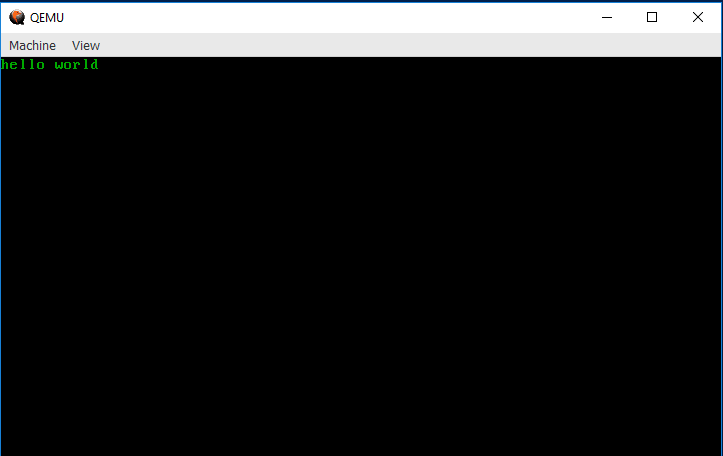# A VGA "hello world"

In the previous chapter, we set up a "hello world" kernel, but we didn't even print "hello world" to the screen! Let's fix that. Open up your `src/main.rs` and modify your `_start()` function to look like this:

``````
# #![allow(unused_variables)]
#fn main() {
#[no_mangle]
pub fn _start() -> ! {
let slice = unsafe { core::slice::from_raw_parts_mut(0xb8000 as *mut u8, 4000) };

slice = b'h';
slice = 0x02;
slice = b'e';
slice = 0x02;
slice = b'l';
slice = 0x02;
slice = b'l';
slice = 0x02;
slice = b'o';
slice = 0x02;
slice = b' ';
slice = 0x02;
slice = b'w';
slice = 0x02;
slice = b'o';
slice = 0x02;
slice = b'r';
slice = 0x02;
slice = b'l';
slice = 0x02;
slice = b'd';
slice = 0x02;

loop {}
}
#}``````

If you compile and build with `bootimage run`, you should see something like this appear!Congrats! You've gotten it to print to the screen. If you look at the code above, you may be getting an idea of why VGA is called "video graphics array"... in the next section, we'll explain what the code above is actually doing.